High energy storage capacitors derivation and applications
2022-12-20

The capacitor is a two-terminal electrical component where two terminals are arranged side by side and separated by an insulator. The main function of a capacitor is to store electrical energy and its common usage mainly includes voltage spike protection, signal filtering & energy storage. But how this energy is stored in a capacitor? So, this article will give you information on how does high energy storage capacitors store the energy and its application.

What is energy stored in capacitor?

A capacitor stores energy in the electrical field between its two plates. When a capacitor is charged through a battery, then an electrical field can be built up. Once a charged capacitor is detached from a battery, then its energy will stay in the field within the gap between its two plates.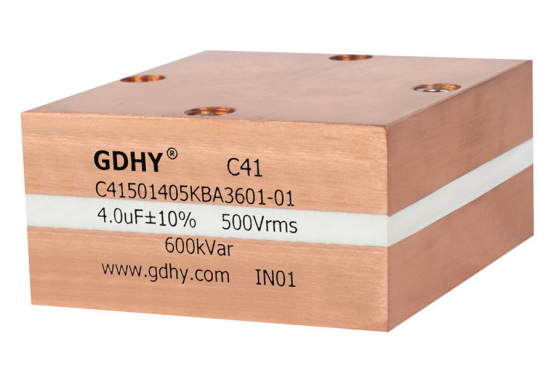Energy stored in capacitor derivation

The energy stored in the capacitor diagram is shown below. Whenever a battery is connected across two plates of a capacitor then the capacitor will be charged which leads to an accumulation of charges on the opposite capacitor plates. When a d.c. voltage is applied across the capacitor, the positive charges get accumulated on one plate and an equal number of negative charges on the other plate. Since the charges are separated by a dielectric medium, they face an electric field which opposes their motion. Thus, they remain stationary on their respective plates only as long as the applied voltage is maintained constant.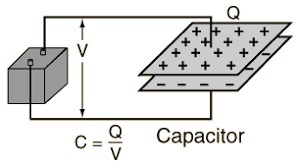Energy stored in the capacitor diagram

Whenever charges build-up, the potential dissimilarity increases slowly across the two capacitor plates. Similarly, when charges are discharged, then the potential dissimilarity can drive a current in the reverse direction.

The energy stored within a capacitor or electric potential energy is related to the charge & voltage on the capacitor. If a conductor’s capacitance is ‘C’, then first it is not charged but gets a potential difference ‘V whenever connected to a battery. If q is the charge on the capacitor plate, then

q = CV

The work done is equivalent to the product of the charge & potential. Thus, W = V*q.

If a small amount of charge is delivered by the battery is ‘dQ’ at a potential ‘V’, and then the work completed is

dW = Vdq = q/c dq

So, the whole work completed in delivering a charge with an amount of ‘q’ toward the capacitor can be given by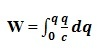W = 1/C [q^2/2] = ½ (q^2/C).

Thus, energy stored within a capacitor is

U = ½ (q^2/C).

Substitute q=CV in the above equation then we can get

U = ½ (CV^2).

Substitute C =q/v in the above equation then we can get

U = ½ qv

The energy stored within a capacitor can be simply expressed in the following ways.

Ecap = QV/2 = CV^2/2 = Q^2/2C

Where,

‘Q’ is the charge

‘V’ is the voltage

‘C’ is the capacitor’s capacitance.

The energy (E) is in joules (J) for a charge (q) in coulombs, voltage (V) in volts & capacitance (C) in farads (F).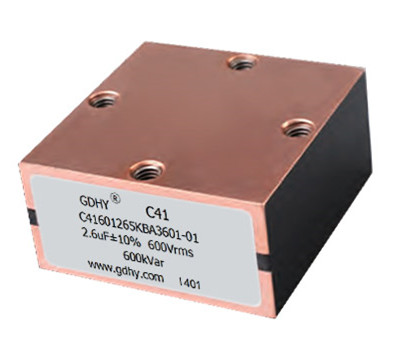Energy stored when capacitors are connected in series

When capacitors like C1, C2 & C3 are connected in series connection with each other respectively then the charge q is given then every capacitor will be charged with q. If C is the net capacity of the series combination.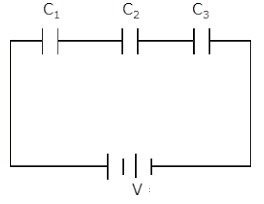Capacitors in series connection

1/C = 1/C1 + 1/C2 + 1/C3

The total energy stored in the series combination is ‘W’

So, W = (1/2) (q^2 / C)

W= (1/2) q^2 [1/ C1 + 1/C2 + 1/ C3 ] => (1/2) q^2 C1 +(1/2) q^2 C2 +(1/2) q^2 C3

Otherwise, the above series combination equation can also be written as;

W = W1 + W2 + W3

Energy stored when capacitors are connected in parallel

When capacitors like C1, C2 & C3 are connected in the parallel combination, then they get charged to a similar potential V. If C is the net capacity of the combination, then,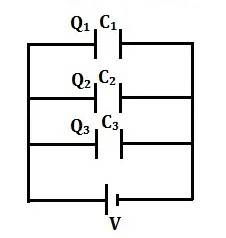Capacitors in parallel connection

C = C1+ C2+C3

The total energy stored in the parallel combination is ‘W’

W = (1/2) ( CV^2 )

W = (1/2)( C1+C2+C3)V^2

Otherwise, the above parallel combination equation can also be written as;

W = W1+W2 +W3

Thus, net energy stored within a combination of capacitors is equivalent to the sum of stored energies within any type of combination of capacitors like series or parallel.

Example1: If a capacitor’s capacitance is 30 F charged to a 100 V potential, then calculate the stored energy in it.

U = ½ CV^2

By substituting the given values in the above equation, we can get

U = ½ 30 (100)^2 = 150 x 10^3 J

Example2: A 12V battery is connected to three capacitors which are connected in series like 10μF, 10μF & 20μF. Calculate the energy stored within the capacitors.

When three capacitors are connected then the capacitance will be

1/C = 1/C1+1/C2+1/C3

1/C = 1/10+1/10+1/20 => 2+2+1/20 =1/4

C= 4uF

The energy stored in the capacitor can be calculated as 1/2CV^2

= 1/2×4 x 10^-6 x (12)^2 = 2x144x10^-6 = 288×10^-6 J

The advantages of energy stored in capacitors include the following:

1. The energy of the capacitor can charge & accumulate very quickly.

2. They can deliver the energy stored rapidly.

3. As compared to other storage devices, losses are less.

4. Less maintenance.

5. Service life is long.

The disadvantages of energy stored in capacitors include the following:

1. As compared to batteries, capacitors have less energy capacity.

2. Energy storage is limited for each dollar cost.

3. The energy stored will reduce eventually because of internal losses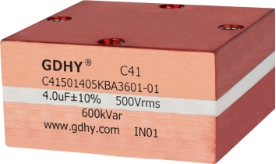Applications

Energy stored in capacitor uses includes the following:

1. Capacitors are used to supply energy to different devices like defibrillators and microelectronics like flash lamps & calculators.

2. Capacitors can emit energy very fast than batteries can which results in much higher power density as compared to batteries with an equal amount of energy.

3. Capacitors are applicable in various electronic devices which use a battery.

4. Capacitors store energy to avoid a memory loss when the battery is being altered.

5. These components play a key role in different practical circuits

6. Moreover, capacitors play a key role in many practical circuits, mainly as current stabilizers and in AC adapters to help in the conversion of AC to DC.

7. Capacitors are resistant to unexpected changes in voltage, so they act as a buffer for electrical energy stored as well as removed to maintain a constant current output. Therefore the capacitor is capable of stabilizing the variable AC & discharge energy at different times.

8. The energy stored in capacitors is applicable in UPS, camera flashes, audio equipment, pulsed loads like lasers, magnetic coils, etc.

9. Supercapacitors are used for storing huge amounts of energy.

Thus this is all about the evolution of energy stored in the capacitor which is the required work to charge the capacitor. So the electrical charge can be stored within the electrical field in the gap between two plates of capacitors.It mainly depends on the amount of charge on the two plates of the capacitor & also on the potential difference between the two plates.

RECOMMENDED FOR YOU
GDHY Revolutionizing Capacitor Design to Enhance Performance and Efficiency in Small Welding and Quenching Equipment
Precautions for using film capacitors
Start capacitor in AC Induction Motor
How does metallized polyester film capacitors work in the application?
How to chose water cooled resonant capacitor ?
What is an axial film capacitor?
What is a plastic film capacitor?
IGBT Snubber Capacitors Selection Guide
What is a metallized polyester film capacitor?
What is a coupling capacitor and a bypass capacitor?
Get In Touch With Us
If you contact us now for more details, you can take samples for free. Our service team will get back to you with in 24 hours normally !